# Slope RR

A line has a rise of 2 and a run of 11. What is the slope?

k =  0.18

### Step-by-step explanation:

$k=\frac{dy}{dx}=\frac{2}{11}=0.18$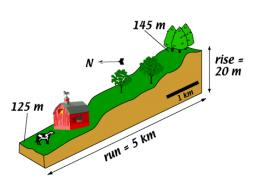Did you find an error or inaccuracy? Feel free to write us. Thank you!Tips to related online calculators
Check out our ratio calculator.

## Related math problems and questions:

• V - slopeThe slope of the line whose equation is -3x -9 = 0 is
• Slope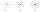Calculate the slope of a line that intersects points (-84,41) and (-76,-32).
• General line equationsIn all examples, write the GENERAL EQUATION OF a line that is given in some way. A) the line is given parametrically: x = - 4 + 2p, y = 2 - 3p B) the line is given by the slope form: y = 3x - 1 C) the line is given by two points: A [3; -3], B [-5; 2] D) t
• Slope of the pool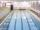Calculate slope (rise:run) of the bottom of the swimming pool long 30 m. Water depth at beginning of the pool is 1.13 m (for children) and depth at the end is 1.84 m (for swimmers). Slope express as percentage and as the angle in degrees.
• SlopeWhat is the slope of the line defined by the equation -2x +3y = -1 ?
• SlopeFind the slope of the line: x=t and y=1+t.
• Line segmentThe 4 cm long line segment is enlarged in the ratio of 5/2. How many centimeters will measure the new line segment?
• Line segmentCut a line segment of 15 cm into two line segments so that their lengths are in ratio 2:1. What length will each have?
• Angles ratioIn a triangle ABC true relationship c is less than b and b is less than a. Internal angles of the triangle are in the ratio 5:4:9. The size of the internal angle beta is:
• SlopeWhat is the slope of a line with an inclination 6.06 rad?
• Perpendicular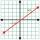What is the slope of the perpendicular bisector of line segment AB if A[9,9] and B[9,-2]?
• Smallest internal angle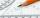Calculate what size has the smallest internal angle of the triangle if values of angles α:β:γ = 3:4:8
• Using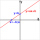Using the point-slope equation, find the equation containing (-7, 3) and slope m = -4
• Points on line segmentPoints P & Q belong to segment AB. If AB=a, AP = 2PQ = 2QB, find the distance: between point A and the midpoint of the segment QB.
• Map 2At what scale is made map if the distance 8.2 km corresponds on the map segment 5 cm long?
• Runner 2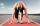Marian runs 12 meters in 8 seconds. How far would Marian run for 70 seconds if he still runs at the same pace?
• Pills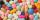If it takes 20 minutes to run a batch of 100 pills how many minutes would it take to run a batch of 50 pills